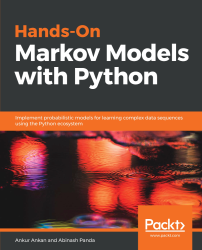•#### Hands-On Markov Models with Python#### Overview of this book

Hidden Markov Model (HMM) is a statistical model based on the Markov chain concept. Hands-On Markov Models with Python helps you get to grips with HMMs and different inference algorithms by working on real-world problems. The hands-on examples explored in the book help you simplify the process flow in machine learning by using Markov model concepts, thereby making it accessible to everyone. Once you’ve covered the basic concepts of Markov chains, you’ll get insights into Markov processes, models, and types with the help of practical examples. After grasping these fundamentals, you’ll move on to learning about the different algorithms used in inferences and applying them in state and parameter inference. In addition to this, you’ll explore the Bayesian approach of inference and learn how to apply it in HMMs. In further chapters, you’ll discover how to use HMMs in time series analysis and natural language processing (NLP) using Python. You’ll also learn to apply HMM to image processing using 2D-HMM to segment images. Finally, you’ll understand how to apply HMM for reinforcement learning (RL) with the help of Q-Learning, and use this technique for single-stock and multi-stock algorithmic trading. By the end of this book, you will have grasped how to build your own Markov and hidden Markov models on complex datasets in order to apply them to projects.
PrefaceFree Chapter
Introduction to the Markov ProcessHidden Markov ModelsState Inference - Predicting the StatesParameter Learning Using Maximum LikelihoodParameter Inference Using the Bayesian ApproachTime Series PredictingNatural Language Processing2D HMM for Image ProcessingMarkov Decision ProcessOther Books You May Enjoy# Random variables

As we always do in statistics, let's start with a simple example of rolling a dice. If we consider rolling a fair dice, the outcome of the dice can be anything from 1 to 6, and is random. To represent such situations (the outcome of rolling the dice in this case), in mathematics we use the concept of random variables. We come across a lot of such variables in our everyday lives. Another example could be ordering food at a restaurant. In this case, the outcome could be any food item on the menu. In general terms, a random variable is a variable whose possible values are outcomes of a random phenomenon. The possible states of the outcomes are also known as the domain of the random variable, and the outcome is based on the probability distribution defined over the domain of the random variable.

Coming back to rolling the dice, the domain of the random variable outcome, O, is given by domain(O) = (1, 2, 3, 4, 5, 6), and the probability distribution is given by a uniform distribution P(o) = 1/6 ∀ ∈ domain(O). Similarly, in the case of the restaurant example, for the random variable choosing a dish, the domain would be every item on the menu, and the probability distribution would depend on your food preference. In both of the previous examples, the domain of the random variable has discrete variables; such random variables are known as discrete random variables. But it's also possible for the domain to be a continuous space. For example, consider the random variable representing the stock price of Google tomorrow. The domain of this random variable will be all positive real numbers with most of the probability mass distributed around ±5% of today's price. Such random variables are known as continuous random variables.